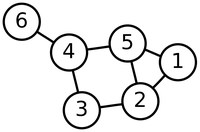# Glossary

## Distance

The distance between two nodes in an (unweighted) graph is the number of edges on the shortest path connecting the two nodes. In a tree, there is only one path between any two nodes, so that the distance between two nodes is simply the number of edges on the unique path connecting them.

For example, consider the unweighted graph below. There are two different paths connecting node 1 to 3, one of which has length 3 and another has length 2, making the distance between the two nodes equal to 2.In the case of a weighted graph, the distance between two nodes is defined as the minimum total weight of any path connecting the nodes, where the total weight of a path is calculated by summing the individual edge weights on the path. Note that in a weighted graph, it is no longer necessarily the case that a minimum-weight path will correspond to a path with a minimum total number of edges.